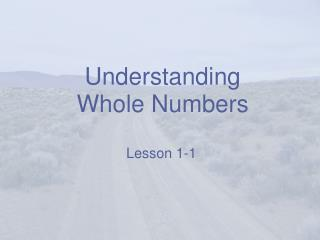DownloadDownload PresentationUnderstanding Whole Numbers

# Understanding Whole Numbers

Télécharger la présentation## Understanding Whole Numbers

- - - - - - - - - - - - - - - - - - - - - - - - - - - E N D - - - - - - - - - - - - - - - - - - - - - - - - - - -
##### Presentation Transcript

1. Understanding Whole Numbers Lesson 1-1

2. Vocabulary standard form – a number is written using digits and place value (the regular way to write numbers). expanded form – a number is written as a sum using the place and value of each digit.

3. Place Value Chart

4. How to Read a Large Number • Numbers are grouped in sets of three (each set is called a period). • Only read three numbers at a time. • Say the name of the period that the numbers are in. • Say “and” for the decimal, but do not say “and” if there isn’t a decimal.

5. Example 4,658,089 • The 4 is in the millions place, and it is the only digit in the millions period. • 658 is in the thousands period (it ends in the thousands place) • 089 is in the ones period. 089 is the same as 89, so we just say “eighty-nine.” Four million, six hundred fifty-eight thousand, eighty nine.

6. Example 2 625,409.36 • 625 is in the thousands period (the 5 is in the thousands place). • 409 is in the ones period. • Now we have a decimal! Say “and” at the decimal. • Now, read the whole number after the decimal. 36 is read “thirty-six.” • What place is the six in? That’s right, the hundredths place. Say “hundredths.” Six hundred twenty-five thousand, four hundred nineandthirty-six hundredths.

7. Expanded Form • Write down the first digit. Fill in zeroes for the rest of the digits in the number. • Place a plus sign (+). • Write down the next digit. Fill in zeroes for all the digits after that number. • Place a plus sign (+). • Repeat until you are done.

8. Example Write 9,987,451,782 in expanded form. + 9 ,000,000,000 + 9 00,000,000 + 8 0,000,000 + 7 ,000,000 + 4 00,000 5 0,000 + 1 ,000 + 7 00 + 8 0 + 2

9. Number Lines Numbers towards the right on a number line are larger. As you move to the left on a number line, the numbers get smaller.

10. What’s Bigger? 1 or -2? 1 is larger because it is to the right of the -2. What numbers are smaller than -2? -3 and -4 are both smaller than -2 because they are to the left of -2.

11. Comparing Numbers • Line up the numbers vertically (up and down) by the ones place (or the decimal, if there is one). • Start at the left and compare the digits. • Move towards the right until you find a difference.

12. Just a Reminder… < means “less than.” > means “greater than.” = means “equal to.”

13. What’s Bigger? 456,789 or 456, 791? Line them up vertically: 456,789 456,791 Now, compare the numbers in the hundred thousands place. They are both 4. Next, we have 5’s. No difference. Then we have 6’s. Also, no difference. Now we are at the hundreds place, and we have a 7 in both numbers. When we get to the tens place, we finally have a difference. 9 is bigger than 8, so the second number is bigger.

14. Put the numbers in order:456; 625; 459; 629; 589 1. Start in the hundreds place. 4 is the smallest number, so we have to decide between 456 and 459. 2. 456 is smaller than 459 because of the difference in the ones place. These are the first two numbers. 3. The next number in the hundreds place is 5, so 589 is the next number. 4. The largest numbers start with 6. 625 is smaller then 629 because of the difference in the ones place. These are the last two numbers. • 456 • 625 • 459 • 629 • 589

15. How do we write it? Separate your numbers with a semicolon (;) to avoid confusion. 456;459;589;625;629 Or, you can use the < symbol. List the numbers in order from smallest to largest with the < symbol between them. 456 < 459 < 589 < 625 < 629

16. It’s Your Turn! Use these strategies on your homework problems! • Commonly Misspelled Numbers: • hundred • thousand • eight • forty • ninety